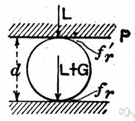# coefficient

(redirected from coefficient of thermal conductivity)
Also found in: Thesaurus, Medical, Acronyms, Encyclopedia.

## co·ef·fi·cient

(kō′ə-fĭsh′ənt)
n.
1. A number or symbol multiplied with a variable or an unknown quantity in an algebraic term, as 4 in the term 4x, or x in the term x(a + b).
2. A numerical measure of a physical or chemical property that is constant for a system under specified conditions, such as the coefficient of friction.

## coefficient

(ˌkəʊɪˈfɪʃənt)
n
1. (Mathematics) maths
a. a numerical or constant factor in an algebraic term: the coefficient of the term 3xyz is 3.
b. the product of all the factors of a term excluding one or more specified variables: the coefficient of x in 3axyz is 3ayz.
2. (General Physics) physics a value that relates one physical quantity to another
[C17: from New Latin coefficiēns, from Latin co- together + efficere to effect]

## co•ef•fi•cient

(ˌkoʊ əˈfɪʃ ənt)

n.
1. a number or quantity placed generally before and multiplying another quantity, as 3 in the expression 3x.
2. Physics. a constant that is a measure of a property of a substance, body, or process: coefficient of friction.
3. acting in consort; cooperating.
[1655–65; < New Latin coefficient-, s. of coefficiēns. See co-, efficient]

## co·ef·fi·cient

(kō′ə-fĭsh′ənt)
A number or symbol multiplied with a variable or an unknown quantity in an algebraic term. For example, 4 is the coefficient in the term 4x, and x is the coefficient in x(a + b).
ThesaurusAntonymsRelated WordsSynonymsLegend:
 Noun 1coefficient - a constant number that serves as a measure of some property or characteristicconstant - a number representing a quantity assumed to have a fixed value in a specified mathematical context; "the velocity of light is a constant"absorptance, absorption coefficient, coefficient of absorption - a measure of the rate of decrease in the intensity of electromagnetic radiation (as light) as it passes through a given substance; the fraction of incident radiant energy absorbed per unit mass or thickness of an absorber; "absorptance equals 1 minus transmittance"coefficient of drag, drag coefficient - the ratio of the drag on a body moving through air to the product of the velocity and the surface area of the bodycoefficient of friction - the ratio of the weight of an object being moved along a surface and the force that maintains contact between the object and the surfacecoefficient of mutual induction, mutual inductance - a measure of the induction between two circuits; the ratio of the electromotive force in a circuit to the corresponding change of current in a neighboring circuit; usually measured in henriescoefficient of self induction, self-inductance - the ratio of the electromotive force produced in a circuit by self-induction to the rate of change of current producing it, expressed in henriesmodulus - (physics) a coefficient that expresses how much of a specified property is possessed by a specified substancecoefficient of expansion, expansivity - the fractional change in length or area or volume per unit change in temperature at a given constant pressurecoefficient of reflection, reflectance, reflection factor, reflectivity - the fraction of radiant energy that is reflected from a surfacetransmittance, transmission - the fraction of radiant energy that passes through a substanceabsolute viscosity, coefficient of viscosity, dynamic viscosity - a measure of the resistance to flow of a fluid under an applied forceweighting, weight - (statistics) a coefficient assigned to elements of a frequency distribution in order to represent their relative importance
Translations
koeficientsoučinitel
koefficient
kerroinmyötävaikuttava
együttható

[ˌkəʊɪˈfɪʃənt] N

[ˌkəʊɪˈfɪʃənt] n

## coefficient

n (Math, Phys) → Koeffizient m

[ˌkəʊɪˈfɪʃnt] n

## co·ef·fi·cient

n. coeficiente, indicación de cambios físicos o químicos producidos por variantes de ciertos factores.
References in periodicals archive ?
The results of predicting the coefficient of thermal conductivity are presented in Figure 10.
Figure 6 plots the ratio of coefficient of thermal conductivity. A higher Ni/Ag nanofluid concentration is associated with higher thermal conductivity, clearly verifying that adding nanoparticles improves the coefficient of thermal conductivity of the working fluid.
The simulation model is necessary to define the thermo-physical properties (specific heat capacity, coefficient of thermal conductivity, bulk density) of the basic and auxiliary materials.

Site: Follow: Share:
Open / Close